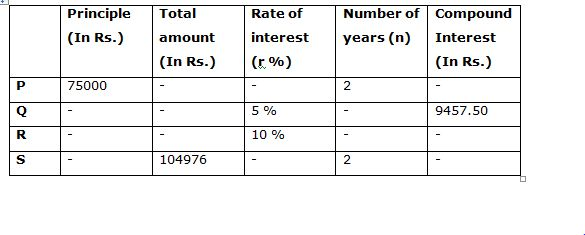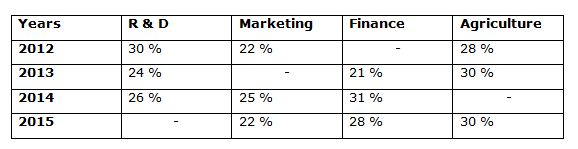# Quantitative Aptitude Questions (Data Interpretation) for SBI Clerk Mains 2018 Day-157

Dear Readers, SBI is conducting Online Examination for the recruitment of clerical cadre To enrich your preparation here we have providing new series of Data Interpretation – Quantitative Aptitude Questions. Candidates those who are appearing in SBI Clerk Mains Exams can practice these Quantitative Aptitude average questions daily and make your preparation effective.

[WpProQuiz 2903]

Click “Start Quiz” to attend these Questions and view Solutions

Directions (1– 5): Study the following information carefully and answer the given questions:

Following table shows the 4 different persons invested the principle amount, total amount and rate of interest and the total number of years they are invested the principle amount and the compound interest for the total investment. (Some values are missing)1) If the interest received by P is Rs. 9270, then find the rate of interest for the principle amount invested by P?

1. 6 %
2. 5 %
3. 8 %
4. 7 %
5. None of these

2) If the total amount received by R is Rs. 73205 and the ratio of the sum invested by P and R is 3: 2, then find the number of years the principle amount invested by R?

1. 2 years
2. 3 years
3. 5 years
4. 4 years
5. None of these

3) If the total amount received by R after investing the principle amount for 4 years is Rs. 92238.30, then find the principle amount invested by R?

1. Rs. 59000
2. Rs. 63000
3. Rs. 65000
4. Rs. 56000
5. None of these

4) If the ratio of the sum invested by P and S is 5: 6, then find the rate of interest for the principle amount invested by S?

1. 5 %
2. 10 %
3. 8 %
4. 6 %
5. None of these

5) If the sum invested by Q is 80 % of the sum invested by P, then find the number of years Q invested the sum?

1. 2 years
2. 4 years
3. 3 years
4. Can’t be determined
5. None of these

Directions (6 – 10) Study the following information carefully and answer the given questions:

Following table shows the percentage distribution of expenses of four departments in 4 different years.Total expenses of these 4 departments

In the year 2012 = 60 lakhs

In the year 2013 = 68 lakhs

In the year 2014 = 72 lakhs

In the year 2015 = 75 lakhs

Note:

Profit = Income – Expense

Profit % = (Profit/Expense)*100

6) If the profit % of Finance department in the year 2012 is 20 %, then find the total income of finance department in the year 2012?

1. 15.2 lakhs
2. 14.4 lakhs
3. 13.8 lakhs
4. 16.4 lakhs
5. None of these

7) Find the difference between the total profit earned by marketing department in the year 2013 to that of total expenses of R & D department in the year 2014 and 2015 together, if the profit % of marketing department in the year 2013 is 15 %?

1. 2895000
2. 2913000
3. 3056000
4. 3117000
5. None of these

8) Find the average expenses of agriculture in all the given years together?

1. 1816500
2. 1578200
3. 1649400
4. 1752600
5. None of these

9) If the profit earned by marketing department and R & D department in the year 2014 and 2015 respectively is 4.5 lakhs and 3 lakhs, then find the profit % of marketing department is what percentage of profit % of R & D department?

1. 110 %
2. 135 %
3. 125 %
4. 100 %
5. 90 %

10) Find the ratio between the total expenses of marketing and agriculture department in the year 2012 to that of total expenses of agriculture department in the year 2014?

1. 125: 54
2. 112: 73
3. 96: 65
4. 88: 47
5. None of these

Compound Interest = Rs. 9270

Total amount = 75000 + 9270 = Rs. 84270

Number of years (n) = 2

Total amount = P*(1 + (r/100))n

84270 = 75000*(1 + (r/100))2

(84270/75000) = [(100 + r)/100]2

(2809/2500) = [(100 + r)/100]2

(53/50)2 = [(100 + r)/100]2

(53/50) = [(100 + r)/100]

106 = 100 + r

r = 6 %

Total amount = Rs. 73205

Ratio of the sum invested by P and R = 3: 2 (3x, 2x)

3x = 75000

X = 25000

Principle amount invested by R = 2x = Rs. 50000

Total amount = P*(1 + (r/100))n

73205 = 50000*[110/100]n

73205/50000 = [11/10]n

14641/10000 = [11/10]n

(11/10)4 = [11/10]n

n = 4

Number of years = 4 years

Total amount = Rs. 92238.30

Total amount = P*(1 + (r/100))n

92238.30 = P*(1 + (10/100))4

92238.30 = P*(110/100)4

92238.30 = P*(11/10)4

P = 92238.30*(10000/14641)

P = Rs. 63000

The ratio of the sum invested by P and S = 5: 6 (5x, 6x)

5x = 75000

X = 15000

The principle amount invested by S = 6x = Rs. 90000

Total amount = Rs. 104976

Total amount = P*(1 + (r/100))n

104976 = 90000*[(100 + r)/100]2

(104976/90000) = [(100 + r)/100]2

(729/625) = [(100 + r)/100]2

(27/25)2 = [(100 + r)/100]2

27/25 = [(100 + r)/100]

108 = 100 + r

r = 8 %

Rate of interest = 8 %

Principle amount invested by Q = (80/100)*75000

= > Rs. 60000

Compound interest = Rs. 9457.50

Total amount = 60000 + 9457.50 = Rs. 69457.50

Total amount = P*(1 + (r/100))n

69457.50 = 60000*(1 + (5/100))n

(69457.50/60000) = (105/100)n

(694575/600000) = (21/20)n

9261/8000 = (21/20)n

(21/20)3 = (21/20)n

n = 3

Number of years = 3 years

Direction (6-10)

Total expenses of 4 departments in the year 2012 = 60 lakhs

Total expenses of finance department in the year 2012

= > 60*(20/100) = 12 lakhs

Profit % = 20 %

Total income of finance department in the year 2012

= > Income = 12*(120/100)

= > Income = 14.4 lakhs

The total profit earned by marketing department in the year 2013

Expense of marketing in the year 2013 = 68*(25/100) = 17 lakhs

Income of marketing in the year 2013 = 17*(115/100) = 19.55 lakhs

Profit = 2.55 lakhs

Total expenses of R & D department in the year 2014 and 2015 together

= > 72*(26/100) + 75*(20/100)

= > 18.72 + 15 = 33.72 lakhs

Required difference = 33.72 – 2.55 = 31.17 lakhs = 3117000

The total expenses of agriculture in all the given years together

= > 60*(28/100) + 68*(30/100) + 72*(18/100) + 75*(30/100)

= > 16.8 + 20.4 + 12.96 + 22.5

= > 72.66 lakhs

Required average = 72.66/4 = 18.165 lakhs = 1816500

The profit earned by marketing department the year 2014 = 4.5 lakhs

Expense of marketing department the year 2014 = 72*(25/100) = 18 lakhs

Profit % of marketing department the year 2014 = (4.5/18)*100 = 25 %

The profit earned by R & D department the year 2015 = 3 lakhs

Expense of R & D department the year 2015 = 75*(20/100) = 15 lakhs

Profit % of R & D department the year 2015 = (3/15)*100 = 20 %

Required % = (25/20)*100 = 125 %

The total expenses of marketing and agriculture department in the year 2012

= > 60*(50/100) = 30 lakhs

The total expenses of agriculture department in the year 2014

= > 72*(18/100) = 12.96 lakhs

Required ratio = 30: 12.96 = 3000: 1296 = 125: 54

Daily Practice Test Schedule | Good Luck

 Topic Daily Publishing Time Daily News Papers & Editorials 8.00 AM Current Affairs Quiz 9.00 AM Quantitative Aptitude “20-20” 11.00 AM Vocabulary (Based on The Hindu) 12.00 PM General Awareness “20-20” 1.00 PM English Language “20-20” 2.00 PM Reasoning Puzzles & Seating 4.00 PM Daily Current Affairs Updates 5.00 PM Data Interpretation / Application Sums (Topic Wise) 6.00 PM Reasoning Ability “20-20” 7.00 PM English Language (New Pattern Questions) 8.00 PM